Nonequispaced fast Fourier transform
Hyperbolic cross
NNFFT

# NSFFT - nonequispaced sparse FFT

In order to circumvent the `curse of dimensionality' in multivariate approximation, interpolations on sparse grids were introduced.

Let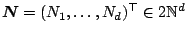,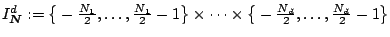, and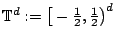. The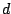-variate NFFT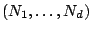computes approximations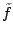of the trigonometric polynomial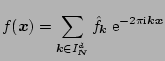at arbitrary knots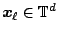,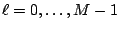.

This library of C functions evaluates the trigonometric polynomial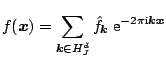at arbitrary knots,and for a hyperbolic cross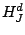which can be partitioned into blocks of indices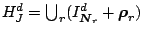with frequency shifts.

Figure: Hyperbolic cross points in 2D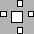Figure: Hyperbolic cross points in 3D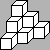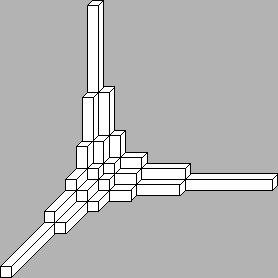The algorithms are implemented by Markus Fenn and Stefan Kunis in ./examples/nsfft. Related paper areFenn, M., Kunis, S., Potts, D.
Fast evaluation of trigonometric polynomials from hyperbolic crosses.
Numer. Algorithms 41, 339-252. (full paper ps, pdf),   2006Döhler, M., Kunis, S. and Potts, D.
Nonequispaced hyperbolic cross fast Fourier transform.
SIAM J. Numer. Anal. 47, 4415 - 4428   (full paper ps, pdf),   2009